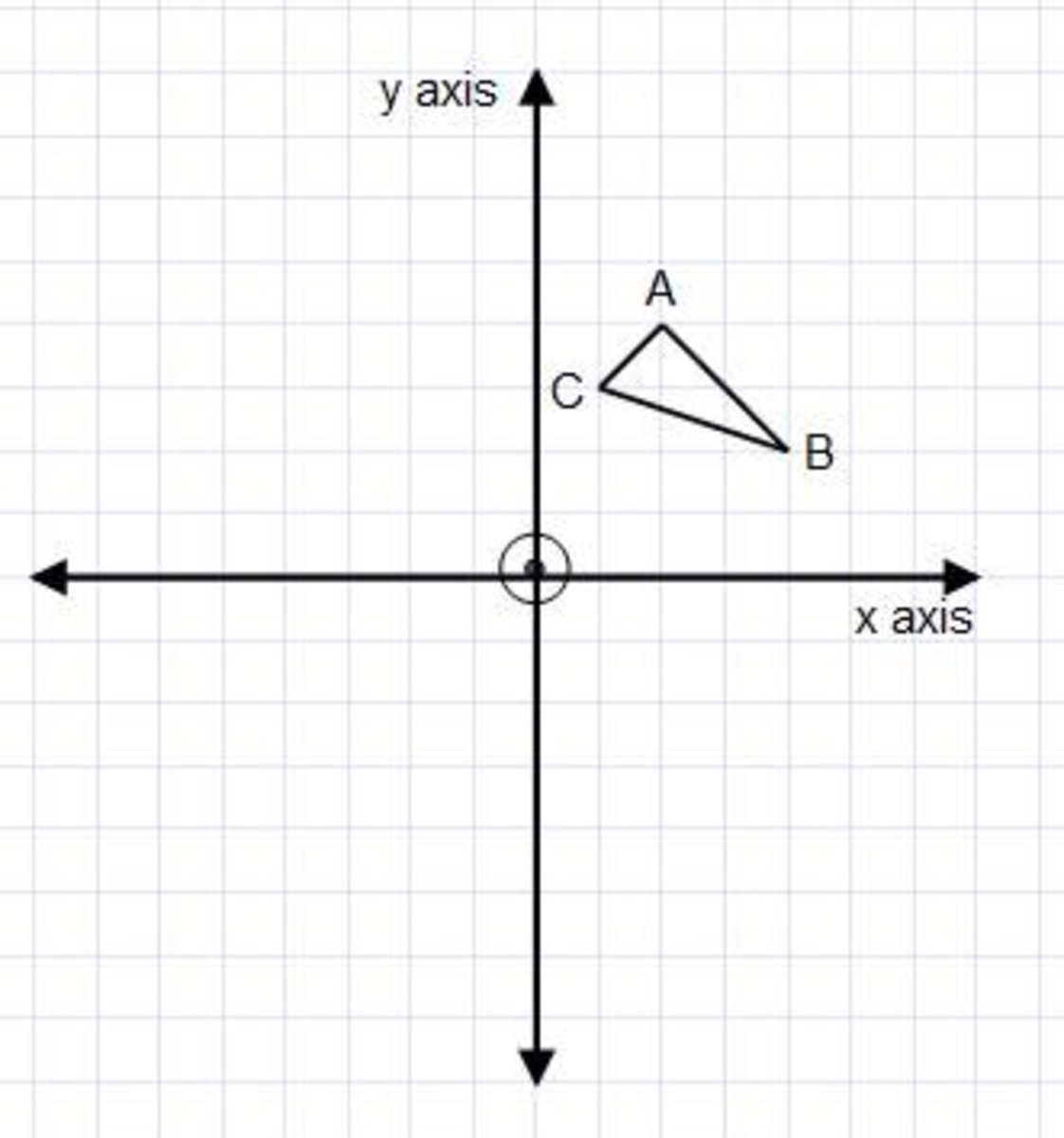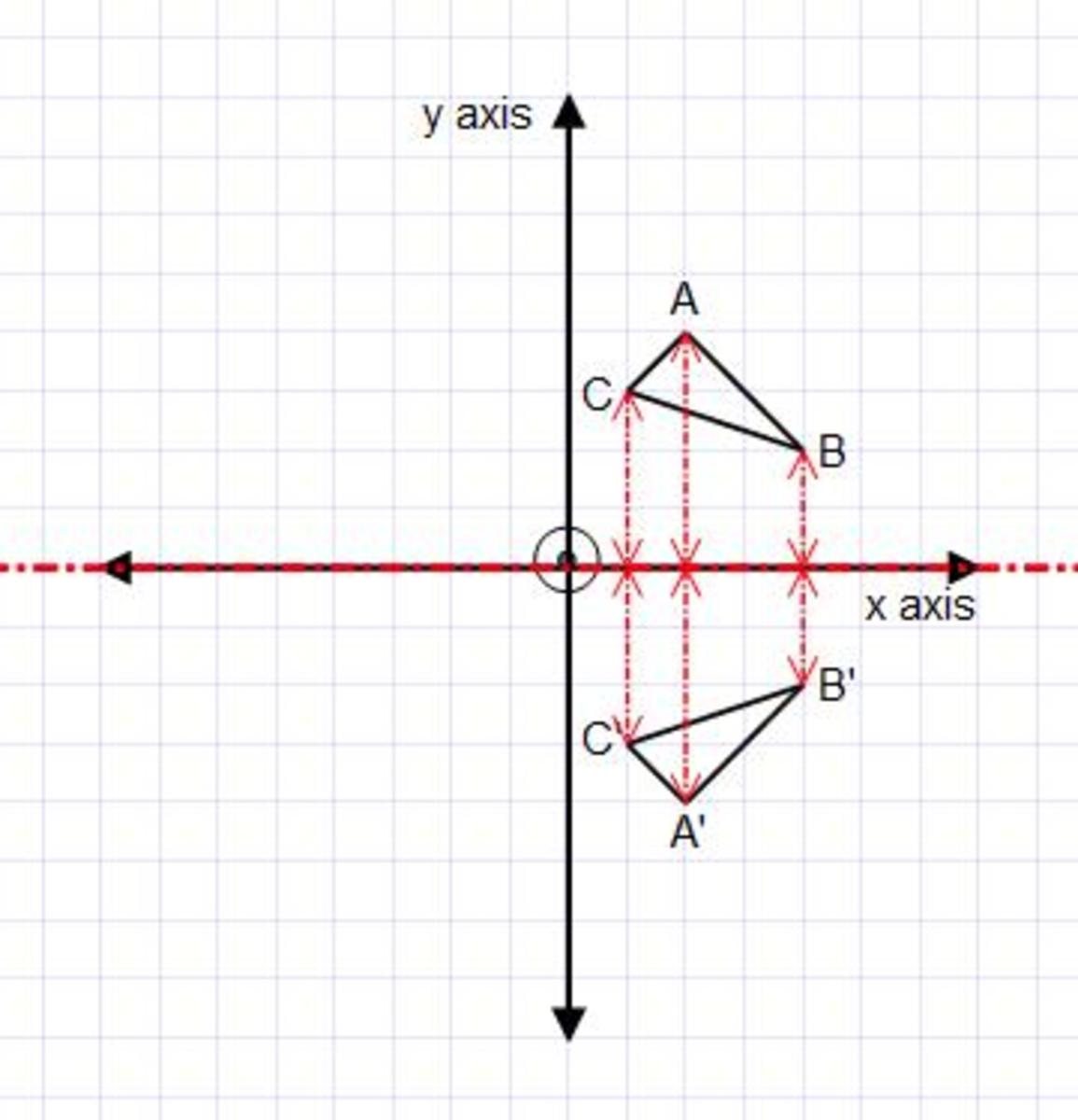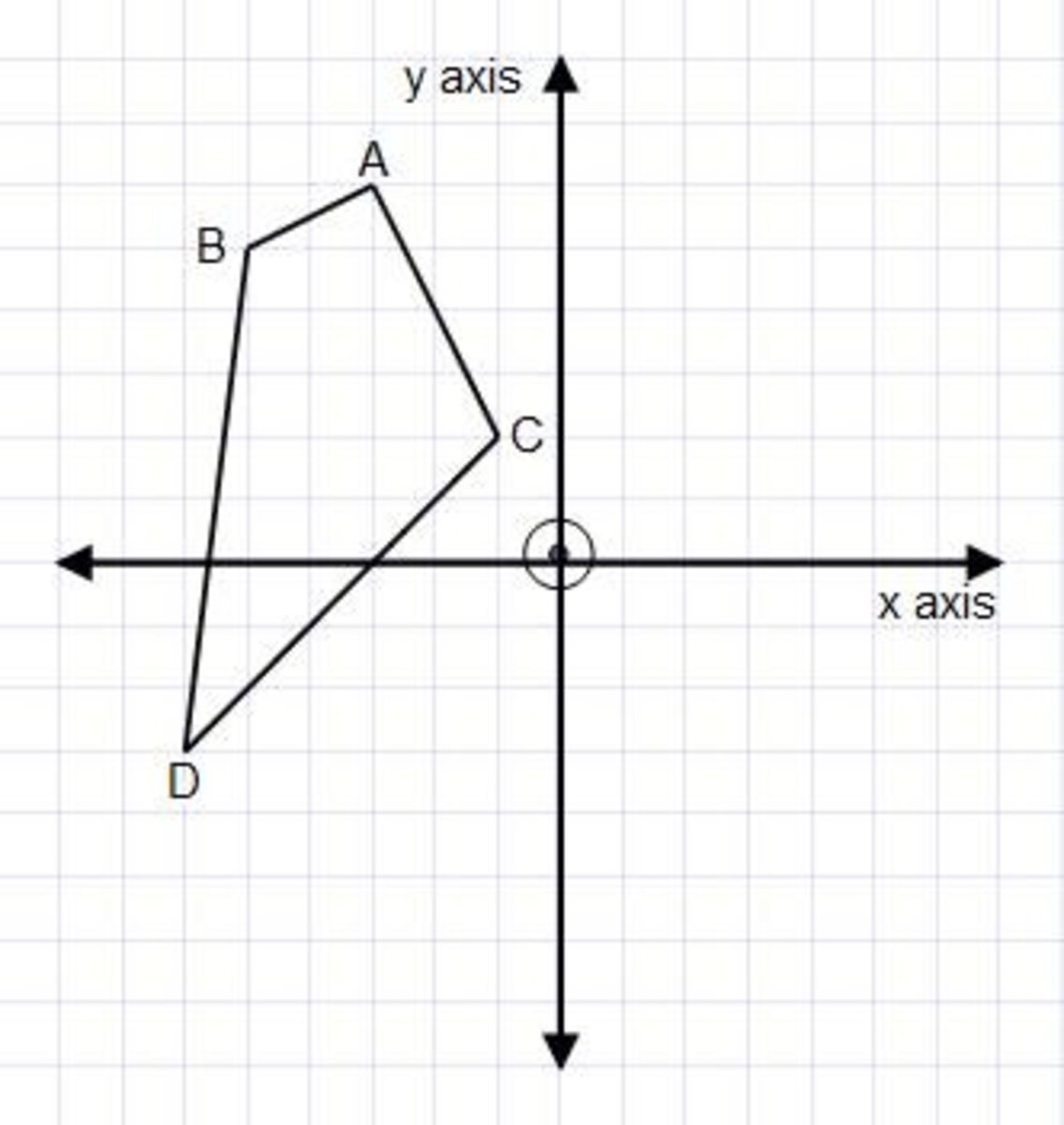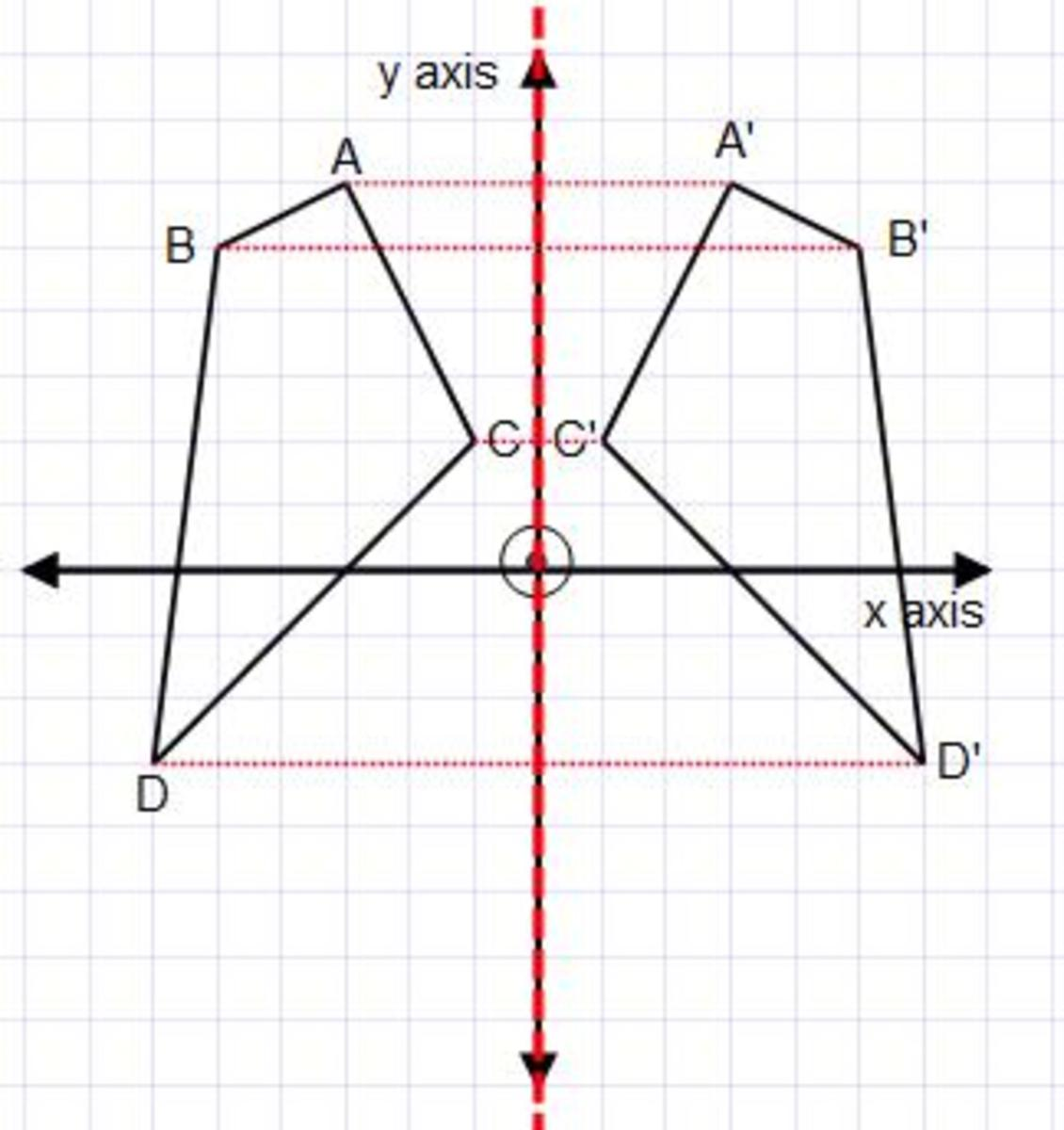Updated date:

# Examples on how to reflect a shape in the x-axis or y-axis on a coordinate grid.

Author:

## How To Reflect Shapes Video

Sometimes you will be asked to reflect a shape on a coordinate grid. If the question asks you to reflect the shape in the x-axis then the x-axis is acting as the mirror line. If the question asks you to reflect the shape in the y-axis then the y-axis is acting as the mirror line.

All you need to do then is measure the distance of each corner of the shape to the x-axis or y-axis (easier to count squares than use a ruler) and then count the same distance away from the x-axis or y-axis. Once the new corners are plotted join them up with your ruler. Check that the reflected shape looks correct as it will be turned the other way round to the original shape.

Example 1Reflect the shape in the x-axis.

First go over the x – axis with a different colour so you know exactly where the mirror line is.

Corner A is 4 squares to the x-axis, so corner A on the reflected shape will be 4 squares away from the x-axis.

Corner B is 2 squares to the x-axis, so corner B on the reflected shape will be 2 squares away from the x-axis.

Corner C is 3 squares to the x-axis, so corner C on the reflected shape will be 3 squares away from the x-axis.

Once you have plotted these three new points then join them up neatly using a mirror.Example 2

Reflect the shape in the y-axis.

First go over the x – axis with a different colour so you know exactly where the mirror line is.

Corner A is 3 squares to the y-axis, so corner A on the reflected shape will be 4 squares away from the y-axis.

Corner B is 5 squares to the y-axis, so corner B on the reflected shape will be 5 squares away from the y-axis.

Corner C is 1 square to the y-axis, so corner C on the reflected shape will be 1 square away from the y-axis.

Corner D is 6 square to the y-axis, so corner D on the reflected shape will be 6 squares away from the y-axis.

Once you have plotted these four new points then join them up neatly using a mirror.